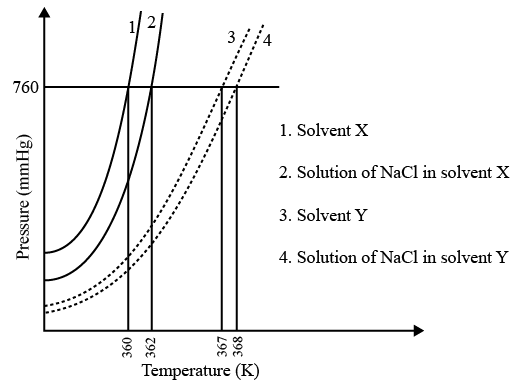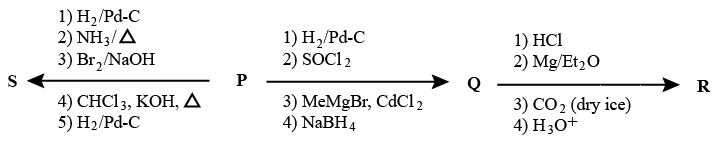Instructions

For each question, enter the correct numerical value (in decimal notation, truncated/rounded-off to the second decimal place; e.g. 6.25, 7.00, -0.33, -.30, 30.27, -127.30) using the mouse and the on-screen virtual numeric keypad in the place designated to enter the answer.

Question 31

Backspace
789
456
123
0.-
Clear All
Question 32

# The plot given below shows 𝑃 − 𝑇 curves (where P is the pressure and T is the temperature) for two solvents X and Y and isomolal solutions of NaCl in these solvents. NaCl completely dissociates in both the solvents.On addition of equal number of moles of a non-volatile solute S in equal amount (in kg) of these solvents, the elevation of boiling point of solvent X is three times that of solvent Y. Solute S is known to undergo dimerization in these solvents. If the degree of dimerization is 0.7 in solvent Y, the degree of dimerization in solvent X is ____.

Backspace
789
456
123
0.-
Clear All
Instructions

Treatment of benzene with $$\frac{CO}{HCl}$$ in the presence of anhydrous $$\frac{AlCl_{3}}{CuCl}$$ followed by reaction with $$\frac{Ac_{2}O}{NaOAc}$$ gives compound X as the major product. Compound X upon reaction with $$\frac{Br_{2}}{Na_{2}CO_{3}}$$, followed by heating at 473 K with moist KOH furnishes Y as the major product. Reaction of X with $$\frac{H_{2}}{Pd-C}$$, followed by $$H_{3}PO_{4}$$ treatment gives Z as the major product.

Question 33

Question 34

# The compound Z is

Instructions

An organic acid P (C11H12O2) can easily be oxidized to a dibasic acid which reacts with ethyleneglycol to produce a polymer dacron. Upon ozonolysis, P gives an aliphatic
ketone as one of the products. P undergoes the following reaction sequences to furnish R via Q. The compound P also undergoes another set of reactions to produce SQuestion 35

Question 36

# The compound S

Instructions

For the following questions answer them individually

Question 37

Question 38

Question 39

Question 40

OR